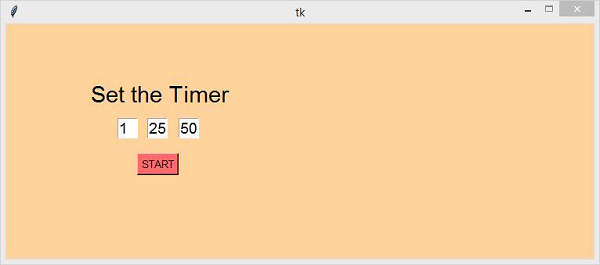# How to create a timer using tkinter?

In this example, we will create a timer using Python Tkinter. For displaying time, we will use the Time Module in Python.

Initially, we will follow these steps to create the timer,

• Create three entry widget each for Hours, Minute and Seconds and set the value ‘00’ by default.
• Create a Button for setting the timer. It will call the function countdowntimer().
• Define a function countdowntimer() which does update once we click on the button to propagate the time.

## Example

#Import the required library
from tkinter import *
import time
#Create an instance of tkinter frame
win = Tk()
win.geometry('750x300')
win.resizable(False,False)
#Configure the background
win.config(bg='burlywood1')
#Create Entry Widgets for HH MM SS
sec = StringVar()
Entry(win, textvariable=sec, width = 2, font = 'Helvetica 14').place(x=220, y=120)
sec.set('00')
mins= StringVar()
Entry(win, textvariable = mins, width =2, font = 'Helvetica
14').place(x=180, y=120)
mins.set('00')
hrs= StringVar()
Entry(win, textvariable = hrs, width =2, font = 'Helvetica 14').place(x=142, y=120)
hrs.set('00')
#Define the function for the timer
def countdowntimer():
times = int(hrs.get())*3600+ int(mins.get())*60 + int(sec.get())
while times > -1:
minute,second = (times // 60 , times % 60)
hour =0
if minute > 60:
hour , minute = (minute // 60 , minute % 60)
sec.set(second)
mins.set(minute)
hrs.set(hour)
#Update the time
win.update()
time.sleep(1)
if(times == 0):
sec.set('00')
mins.set('00')
hrs.set('00')
times -= 1
Label(win, font =('Helvetica bold',22), text = 'Set the Timer',bg
='burlywood1').place(x=105,y=70)
Button(win, text='START', bd ='2', bg = 'IndianRed1',font =('Helvetica
bold',10), command = countdowntimer).place(x=167, y=165)
win.mainloop()

## Output

The above code will display a clock timer.Now, set the timer by inserting some value in the box and click the "START" button to start the timer.